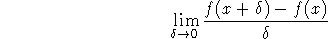Next: Bracketing Up: Commands for Math Mode Previous: Sequences of Equations

## Getting Stuff On Top of Other Stuff

If you want to make a fraction, use the \frac command; \frac works on everything contained in a pair of curly brackets, or the entire equation if there is no enclosing pair of curly brackets.

Here's an example:

$$\lim_{\delta\rightarrow 0}\frac{f(x+\delta) - f(x)}{\delta}$$
givesProcessed by LaTeX2html